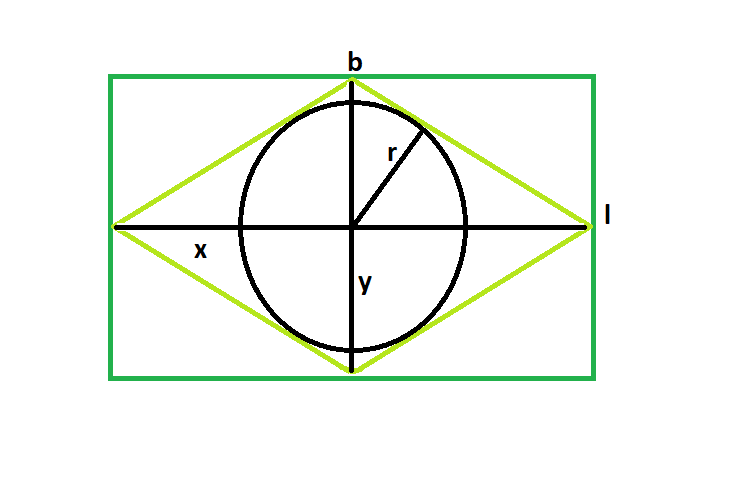# Radius of the biggest possible circle inscribed in rhombus which in turn is inscribed in a rectangle

Give a rectangle with length l & breadth b, which inscribes a rhombus, which in turn inscribes a circle. The task is to find the radius of this circle.

Examples:

```Input: l = 5, b = 3
Output: 1.28624

Input: l = 6, b = 4
Output: 1.6641

```

## Recommended: Please try your approach on {IDE} first, before moving on to the solution.Approach: From the figure, it is clear that diagonals, x & y, are equal to the length and breadth of the rectangle.
Also radius of the circle, r, inside a rhombus is = xy/2√(x^2+y^2).
So, radius of the circle in terms of l & b is = lb/2√(l^2+b^2).

Below is the implementation of the above approach:

## C++

 `// C++ implementation of above approach ` `#include ` `using` `namespace` `std; ` ` `  `// Function to find the radius ` `// of the inscribed circle ` `float` `circleradius(``float` `l, ``float` `b) ` `{ ` ` `  `    ``// the sides cannot be negative ` `    ``if` `(l < 0 || b < 0) ` `        ``return` `-1; ` ` `  `    ``// radius of the circle ` `    ``float` `r = (l * b) / (2 * ``sqrt``((``pow``(l, 2) + ``pow``(b, 2)))); ` `    ``return` `r; ` `} ` ` `  `// Driver code ` `int` `main() ` `{ ` `    ``float` `l = 5, b = 3; ` `    ``cout << circleradius(l, b) << endl; ` ` `  `    ``return` `0; ` `} `

## Java

 `// Java implementation of above approach ` ` `  `import` `java.io.*; ` ` `  `class` `GFG { ` `     `  `// Function to find the radius ` `// of the inscribed circle ` `static` `float` `circleradius(``float` `l, ``float` `b) ` `{ ` ` `  `    ``// the sides cannot be negative ` `    ``if` `(l < ``0` `|| b < ``0``) ` `        ``return` `-``1``; ` ` `  `    ``// radius of the circle ` `    ``float` `r = (``float``)((l * b) / (``2` `* Math.sqrt((Math.pow(l, ``2``) + Math.pow(b, ``2``))))); ` `    ``return` `r; ` `} ` ` `  `    ``// Driver code ` `    ``public` `static` `void` `main (String[] args) { ` `        ``float` `l = ``5``, b = ``3``; ` `    ``System.out.print (circleradius(l, b)) ; ` `    ``} ` `} ` `// This code is contributed by inder_verma.. `

## Python3

 `# Python 3 implementation of  ` `# above approach ` `from` `math ``import` `sqrt ` ` `  `# Function to find the radius ` `# of the inscribed circle ` `def` `circleradius(l, b): ` `     `  `    ``# the sides cannot be negative ` `    ``if` `(l < ``0` `or` `b < ``0``): ` `        ``return` `-``1` ` `  `    ``# radius of the circle ` `    ``r ``=` `(l ``*` `b) ``/` `(``2` `*` `sqrt((``pow``(l, ``2``) ``+`  `                             ``pow``(b, ``2``)))); ` `    ``return` `r ` ` `  `# Driver code ` `if` `__name__ ``=``=` `'__main__'``: ` `    ``l ``=` `5` `    ``b ``=` `3` `    ``print``(``"{0:.5}"` `. ``format``(circleradius(l, b))) ` ` `  `# This code is contribute  ` `# by Surendra_Gagwar `

## C#

 `// C# implementation of above approach ` `using` `System; ` ` `  `class` `GFG  ` `{ ` `     `  `// Function to find the radius ` `// of the inscribed circle ` `static` `float` `circleradius(``float` `l, ` `                          ``float` `b) ` `{ ` ` `  `    ``// the sides cannot be negative ` `    ``if` `(l < 0 || b < 0) ` `        ``return` `-1; ` ` `  `    ``// radius of the circle ` `    ``float` `r = (``float``)((l * b) / ` `              ``(2 * Math.Sqrt((Math.Pow(l, 2) + ` `                   ``Math.Pow(b, 2))))); ` `    ``return` `r; ` `} ` ` `  `// Driver code ` `public` `static` `void` `Main ()  ` `{ ` `    ``float` `l = 5, b = 3; ` `    ``Console.WriteLine(circleradius(l, b)); ` `} ` `} ` ` `  `// This code is contributed ` `// by inder_verma `

## PHP

 ` `

Output:

```1.28624
```

My Personal Notes arrow_drop_upCheck out this Author's contributed articles.

If you like GeeksforGeeks and would like to contribute, you can also write an article using contribute.geeksforgeeks.org or mail your article to contribute@geeksforgeeks.org. See your article appearing on the GeeksforGeeks main page and help other Geeks.

Please Improve this article if you find anything incorrect by clicking on the "Improve Article" button below.